Lesson Video:

This article is also a Jupyter Notebook available to be run from the top down. There will be code snippets that you can then run in any environment.

Below are the versions of fastai, fastcore, and wwf currently running at the time of writing this:

• fastai: 2.1.10
• fastcore: 1.3.13
• wwf: 0.0.5

Grab our vision related libraries

from fastai.vision.all import *

Below you will find the exact imports for everything we use today

from torch import nn

from fastai.callback.hook import summary
from fastai.callback.schedule import fit_one_cycle, lr_find
from fastai.callback.progress import ProgressCallback

from fastai.data.core import Datasets, DataLoaders, show_at
from fastai.data.external import untar_data, URLs
from fastai.data.transforms import Categorize, GrandparentSplitter, parent_label, ToTensor, IntToFloatTensor, Normalize

from fastai.layers import Flatten
from fastai.learner import Learner

from fastai.metrics import accuracy, CrossEntropyLossFlat

from fastai.vision.core import PILImageBW
from fastai.vision.utils import get_image_files

And our data

path = untar_data(URLs.MNIST)

Working with the data

items = get_image_files(path)
items
Path('/root/.fastai/data/mnist_png/testing/3/4044.png')

Create an image object. Done automatically with ImageBlock.

im = PILImageBW.create(items)
im.show()
<matplotlib.axes._subplots.AxesSubplot at 0x7f0dd07260b8>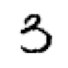Split our data with GrandparentSplitter, which will make use of a train and valid folder.

splits = GrandparentSplitter(train_name='training', valid_name='testing')
items[:3]
(#3) [Path('/root/.fastai/data/mnist_png/testing/3/4044.png'),Path('/root/.fastai/data/mnist_png/testing/3/1205.png'),Path('/root/.fastai/data/mnist_png/testing/3/3916.png')]

Splits need to be applied to some items

splits = splits(items)
splits[:5], splits[:5]
([10000, 10001, 10002, 10003, 10004], [0, 1, 2, 3, 4])
• Make a Datasets

• Expects items, transforms for describing our problem, and a splitting method

dsrc = Datasets(items, tfms=[[PILImageBW.create], [parent_label, Categorize]],
splits=splits)

We can look at an item in our Datasets with show_at

show_at(dsrc.train, 3)
<matplotlib.axes._subplots.AxesSubplot at 0x7f0dd06abef0>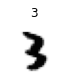We can see that it's a PILImage of a three, along with a label of 3

Next we need to give ourselves some transforms on the data! These will need to:

1. Ensure our images are all the same size
2. Make sure our output are the tensor our models are wanting
3. Give some image augmentation
gpu_tfms = [IntToFloatTensor(), Normalize()]

And show a batch

dls.show_batch()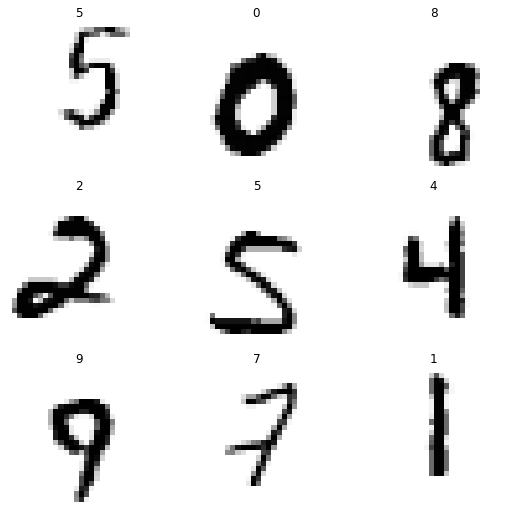From here we need to see what our model will expect

xb, yb = dls.one_batch()

And now the shapes:

xb.shape, yb.shape
((128, 1, 28, 28), (128,))
dls.c
10

So our input shape will be a [128 x 1 x 28 x 28] and our output shape will be a  tensor that we need to condense into 10 classes

The Model

Our models are made up of layers, and each layer represents a matrix multiplication to end up with our final y. For this image problem, we will use a Convolutional layer, a Batch Normalization layer, an Activation Function, and a Flattening layer

Convolutional Layer

These are always the first layer in our network. I will be borrowing an analogy from here by Adit Deshpande.

Our example Convolutional layer will be 5x5x1

Imagine a flashlight that is shining over the top left of an image, which covers a 5x5 section of pixels at one given moment. This flashlight then slides crosses our pixels at all areas in the picture. This flashlight is called a filter, which can also be called a neuron or kernel. The region it is currently looking over is called a receptive field. This filter is also an array of numbers called weights (or parameters). The depth of this filter must be the same as the depth of our input. In our case it is 1 (in a color image this is 3). Now once this filter begins moving (or convolving) around the image, it is multiplying the values inside this filter with the original pixel value of our image (also called element wise multiplications). These are then summed up (in our case this is just one multiplication of 28x28) to an individual value, which is a representation of just the top left of our image. Now repeat this until every unique location has a number and we will get what is called an activation or feature map. This feature map will be 784 different locations, which turns into a 28x28 array

def conv(ni, nf): return nn.Conv2d(ni, nf, kernel_size=3, stride=2, padding=1)

Here we can see our ni is equivalent to the depth of the filter, and nf is equivalent to how many filters we will be using. (Fun fact this always has to be divisible by the size of our image).

Batch Normalization

As we send our tensors through our model, it is important to normalize our data throughout the network. Doing so can allow for a much larger improvement in training speed, along with allowing each layer to learn independantly (as each layer is then re-normalized according to it's outputs)

def bn(nf): return nn.BatchNorm2d(nf)

nf will be the same as the filter output from our previous convolutional layer

Activation functions

They give our models non-linearity and work with the weights we mentioned earlier along with a bias through a process called back-propagation. These allow our models to learn and perform more complex tasks because they can choose to fire or activate one of those neurons mentioned earlier. On a simple sense, let's look at the ReLU activation function. It operates by turning any negative values to zero, as visualized below: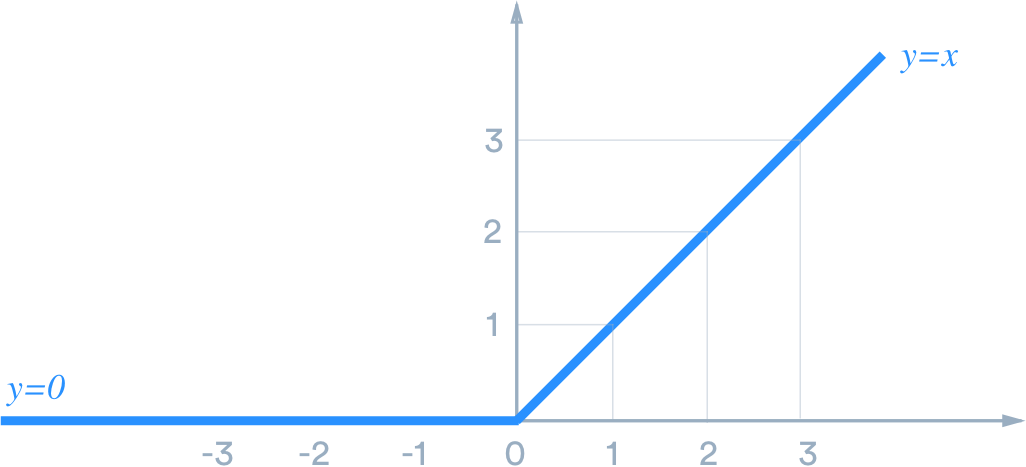From "A Practical Guide to ReLU by Danqing Liu URL.

def ReLU(): return nn.ReLU(inplace=False)

Flattening

The last bit we need to do is take all these activations and this outcoming matrix and flatten it into a single dimention of predictions. We do this with a Flatten() module

Flatten??

Making a Model

• Five convolutional layers
• nn.Sequential
• 1 -> 32 -> 10
model = nn.Sequential(
conv(1, 8),
bn(8),
ReLU(),
conv(8, 16),
bn(16),
ReLU(),
conv(16,32),
bn(32),
ReLU(),
conv(32, 16),
bn(16),
ReLU(),
conv(16, 10),
bn(10),
Flatten()
)

Now let's make our Learner

learn = Learner(dls, model, loss_func=CrossEntropyLossFlat(), metrics=accuracy)

We can then also call learn.summary to take a look at all the sizes with thier exact output shapes

learn.summary()
Sequential (Input shape: 128)
============================================================================
Layer (type)         Output Shape         Param #    Trainable
============================================================================
128 x 8 x 14 x 14
Conv2d                                    80         True
BatchNorm2d                               16         True
ReLU
____________________________________________________________________________
128 x 16 x 7 x 7
Conv2d                                    1168       True
BatchNorm2d                               32         True
ReLU
____________________________________________________________________________
128 x 32 x 4 x 4
Conv2d                                    4640       True
BatchNorm2d                               64         True
ReLU
____________________________________________________________________________
128 x 16 x 2 x 2
Conv2d                                    4624       True
BatchNorm2d                               32         True
ReLU
____________________________________________________________________________
128 x 10 x 1 x 1
Conv2d                                    1450       True
BatchNorm2d                               20         True
____________________________________________________________________________
[]
Flatten
____________________________________________________________________________

Total params: 12,126
Total trainable params: 12,126
Total non-trainable params: 0

Optimizer used: <function Adam at 0x7f280c3f3ea0>
Loss function: FlattenedLoss of CrossEntropyLoss()

Callbacks:
- TrainEvalCallback
- Recorder
- ProgressCallback

learn.summary also tells us:

• Total parameters
• Trainable parameters
• Optimizer
• Loss function
• Applied Callbacks
learn.lr_find()
SuggestedLRs(lr_min=0.33113112449646, lr_steep=0.3019951581954956)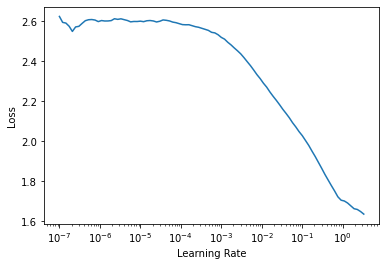Let's use a learning rate around 1e-1 (0.1)

learn.fit_one_cycle(3, lr_max=1e-1)
epoch train_loss valid_loss accuracy time
0 0.221976 0.197778 0.935200 01:32
1 0.121564 0.066874 0.979900 01:33
2 0.068036 0.039550 0.987700 01:32

Simplify it

• Try to make it more like ResNet.
• ConvLayer contains a Conv2d, BatchNorm2d, and an activation function
def conv2(ni, nf): return ConvLayer(ni, nf, stride=2)

And make a new model

net = nn.Sequential(
conv2(1,8),
conv2(8,16),
conv2(16,32),
conv2(32,16),
conv2(16,10),
Flatten()
)

Great! That looks much better to read! Let's make sure we get (roughly) the same results with it.

learn = Learner(dls, net, loss_func=CrossEntropyLossFlat(), metrics=accuracy)
learn.fit_one_cycle(3, lr_max=1e-1)
epoch train_loss valid_loss accuracy time
0 0.220734 0.197168 0.933900 01:15
1 0.130333 0.075714 0.975800 01:14
2 0.078764 0.041104 0.987000 01:15

Almost the exact same! Perfect! Now let's get a bit more advanced

ResNet (kinda)

The ResNet architecture is built with what are known as ResBlocks. Each of these blocks consist of two ConvLayers that we made before, where the number of filters do not change. Let's generate these layers.

class ResBlock(Module):
def __init__(self, nf):
self.conv1 = ConvLayer(nf, nf)
self.conv2 = ConvLayer(nf, nf)

def forward(self, x): return x + self.conv2(self.conv1(x))
• Class notation
• __init__
• foward

Let's add these in between each of our conv2 layers of that last model.

net = nn.Sequential(
conv2(1,8),
ResBlock(8),
conv2(8,16),
ResBlock(16),
conv2(16,32),
ResBlock(32),
conv2(32,16),
ResBlock(16),
conv2(16,10),
Flatten()
)
net
Sequential(
(0): ConvLayer(
(0): Conv2d(1, 8, kernel_size=(3, 3), stride=(2, 2), padding=(1, 1), bias=False)
(1): BatchNorm2d(8, eps=1e-05, momentum=0.1, affine=True, track_running_stats=True)
(2): ReLU()
)
(1): ResBlock(
(conv1): ConvLayer(
(0): Conv2d(8, 8, kernel_size=(3, 3), stride=(1, 1), padding=(1, 1), bias=False)
(1): BatchNorm2d(8, eps=1e-05, momentum=0.1, affine=True, track_running_stats=True)
(2): ReLU()
)
(conv2): ConvLayer(
(0): Conv2d(8, 8, kernel_size=(3, 3), stride=(1, 1), padding=(1, 1), bias=False)
(1): BatchNorm2d(8, eps=1e-05, momentum=0.1, affine=True, track_running_stats=True)
(2): ReLU()
)
)
(2): ConvLayer(
(0): Conv2d(8, 16, kernel_size=(3, 3), stride=(2, 2), padding=(1, 1), bias=False)
(1): BatchNorm2d(16, eps=1e-05, momentum=0.1, affine=True, track_running_stats=True)
(2): ReLU()
)
(3): ResBlock(
(conv1): ConvLayer(
(0): Conv2d(16, 16, kernel_size=(3, 3), stride=(1, 1), padding=(1, 1), bias=False)
(1): BatchNorm2d(16, eps=1e-05, momentum=0.1, affine=True, track_running_stats=True)
(2): ReLU()
)
(conv2): ConvLayer(
(0): Conv2d(16, 16, kernel_size=(3, 3), stride=(1, 1), padding=(1, 1), bias=False)
(1): BatchNorm2d(16, eps=1e-05, momentum=0.1, affine=True, track_running_stats=True)
(2): ReLU()
)
)
(4): ConvLayer(
(0): Conv2d(16, 32, kernel_size=(3, 3), stride=(2, 2), padding=(1, 1), bias=False)
(1): BatchNorm2d(32, eps=1e-05, momentum=0.1, affine=True, track_running_stats=True)
(2): ReLU()
)
(5): ResBlock(
(conv1): ConvLayer(
(0): Conv2d(32, 32, kernel_size=(3, 3), stride=(1, 1), padding=(1, 1), bias=False)
(1): BatchNorm2d(32, eps=1e-05, momentum=0.1, affine=True, track_running_stats=True)
(2): ReLU()
)
(conv2): ConvLayer(
(0): Conv2d(32, 32, kernel_size=(3, 3), stride=(1, 1), padding=(1, 1), bias=False)
(1): BatchNorm2d(32, eps=1e-05, momentum=0.1, affine=True, track_running_stats=True)
(2): ReLU()
)
)
(6): ConvLayer(
(0): Conv2d(32, 16, kernel_size=(3, 3), stride=(2, 2), padding=(1, 1), bias=False)
(1): BatchNorm2d(16, eps=1e-05, momentum=0.1, affine=True, track_running_stats=True)
(2): ReLU()
)
(7): ResBlock(
(conv1): ConvLayer(
(0): Conv2d(16, 16, kernel_size=(3, 3), stride=(1, 1), padding=(1, 1), bias=False)
(1): BatchNorm2d(16, eps=1e-05, momentum=0.1, affine=True, track_running_stats=True)
(2): ReLU()
)
(conv2): ConvLayer(
(0): Conv2d(16, 16, kernel_size=(3, 3), stride=(1, 1), padding=(1, 1), bias=False)
(1): BatchNorm2d(16, eps=1e-05, momentum=0.1, affine=True, track_running_stats=True)
(2): ReLU()
)
)
(8): ConvLayer(
(0): Conv2d(16, 10, kernel_size=(3, 3), stride=(2, 2), padding=(1, 1), bias=False)
(1): BatchNorm2d(10, eps=1e-05, momentum=0.1, affine=True, track_running_stats=True)
(2): ReLU()
)
(9): Flatten()
)

Awesome! We're building a pretty substantial model here. Let's try to make it even simpler. We know we call a convolutional layer before each ResBlock and they all have the same filters, so let's make that layer!

def conv_and_res(ni, nf): return nn.Sequential(conv2(ni, nf), ResBlock(nf))
net = nn.Sequential(
conv_and_res(1,8),
conv_and_res(8,16),
conv_and_res(16,32),
conv_and_res(32,16),
conv2(16,10),
Flatten()
)

And now we have something that resembles a ResNet! Let's see how it performs

learn = Learner(dls, net, loss_func=CrossEntropyLossFlat(), metrics=accuracy)
learn.lr_find()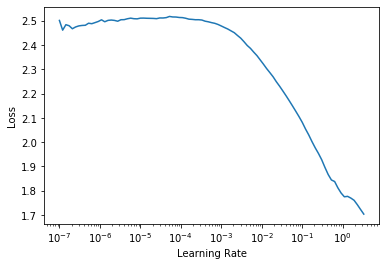Let's do 1e-1 again

learn.fit_one_cycle(3, lr_max=1e-1)
epoch train_loss valid_loss accuracy time
0 0.156825 0.904546 0.756200 01:16
1 0.089813 0.063087 0.980300 01:16
2 0.040906 0.025675 0.992200 01:18